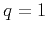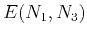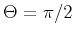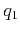On anelliptic approximations for qP velocities in TI and orthorhombic mediaNext: Previous Approximations Up: Transversely isotropic media Previous: Exact Expression

## Muir and Dellinger Approximations

Similar to the derivations by Fomel (2004), the Muir-Dellinger approximations (Muir and Dellinger, 1985; Dellinger et al., 1993) serve as the starting point of our derivation. The Muir-Dellinger phase-velocity approximation is of the following form:(12)

whereis the anelliptic parameter (in case of elliptical anisotropy),denotes the horizontal () velocity squared,denotes the vertical () velocity squared, anddescribes the elliptical part of the velocity and is defined by(13)

The group-velocity approximation takes a similar form, but with symmetric changes in the coefficients and variables,(14)

where,,is group angle (from vertical),denotes the horizontal slowness squared,denotes the vertical slowness squared,, anddescribes the elliptical part of the slowness and is defined by(15)

As suggested by Muir and Dellinger (1985), theparameter can be found by fitting the phase-velocity curvature around either the vertical axis () or the horizontal axis (). The explicit expressions offitting in those two cases are given in equations 1 and 2. If we definein equation 14 by fitting the group velocity curvature around eitheror, we find that(16)

Extending this idea, Dellinger et al. (1993) proposed four-parameter approximations for phase and group velocites using bothand.On anelliptic approximations for qP velocities in TI and orthorhombic mediaNext: Previous Approximations Up: Transversely isotropic media Previous: Exact Expression

2017-04-14